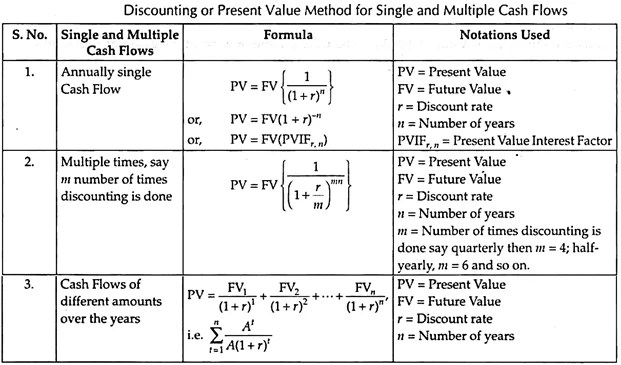# Techniques of Time Value of Money

8th May 2021

Discounting or Present Value Method

The current value of an expected amount of money to be received at a future date is known as Present Value. If we expect a certain sum of money after some years at a specific interest rate, then by discounting the Future Value we can calculate the amount to be invested today, i.e., the current or Present Value.

Hence, Discounting Technique is the method that converts Future Value into Present Value. The amount calculated by Discounting Technique is the Present Value and the rate of interest is the discount rate.Compounding or Future Value Method

Compounding is just the opposite of discounting. The process of converting Present Value into Future Value is known as compounding.

Future Value of a sum of money is the expected value of that sum of money invested after n number of years at a specific compound rate of interest.Differences:

The points of differences between compounding and discounting are as follows:

Difference # Compounding

• The process of converting the Present Value into Future Value is known as compounding.
• Interest rate is used to calculate the Future Value or the compounded value.
• Higher the interest rate greater will be the future or the compounded value.

Future Value is always greater than the Present Value provided the interest rate is positive –

FV = PV (1 + r)n

Difference # Discounting

• The process of converting Future Value in Present Value terms is known as discounting.
• Discount rate is used to calculate the Present Value.
• Higher the discount rate lower will be the Present Value.
• Present Value is always less than the Future Value –

Every finance manager has to take three important Financial Management decisions such as – the Investment Decision, Financing decision and the Dividend decision. Finance manager has to take all these decisions keeping in mind the value maximization or the wealth maximization objective of Financial Management.

So a finance manager before taking a financial decision should keep in mind the objective of value maximization. In case of an Investment Decision, where return is fixed and assured it is said to be risk-free investment, for example, 10% Reserve Bank of India Bonds, government bonds, keeping money in deposit accounts offered by public sector banks, etc. In this case, the probability of the return is 1 and hence no risk is associated with it.

Again for taking Investment Decisions for investing in shares of a company, the risk associated with mutual funds needs to be considered as in this type of investment the return varies which is neither fixed nor assured.

Share market is volatile and the return varies depending on certain macro level factors and company-specific factors such as capital depreciation, fluctuating dividend rate due to profit fluctuation, fund crisis, etc.

So, two aspects are involved in any financial decision one the risk and another return. Both these two factors vary from one decision to another. A proper balance or trade-off between risk and return is required to maximize the return by minimizing the risk and, thereby, achieving the goal of maximizing the market value to shares.